# Rational Numbers As Quotients of Integers

##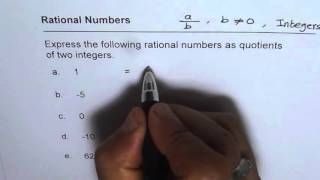By Anil Kumar

every quotient of integers is a rational number.# Ms. Chang 4th Grade- Divide with partial quotients

##By Ms Chang

Find whole-number quotients and remainders with up to four-digit dividends and one-digit divisors, using strategies based on place value, the properties of operations, and/or the relationship between multiplication and division# Four-Digit by One Digit Division (Equal Groups/Partial Quotients): Grade 4

##By University Of Houstan Mathematics Education

Find whole-number quotients and remainders with up to four-digit dividends and one-digit divisors, using strategies based on place value# Complex Numbers In Polar Form De Moivre's Theorem, Products, Quotients, Powers, and nth Roots Prec

##By The Organic Chemistry Tutor

This precalculus video tutorial focuses on complex numbers in polar form and de moivre's theorem. It explains how to find the products, quotients, powers and nth roots of complex numbers in polar form as well as converting it to and from rectangular form. This video contains plenty of examples and practice problems and is useful for high school and college students taking precalculus or trigonometry. Here is a list of topics: 1. Graphing / Plotting Complex Numbers in a Complex Plane 2. Real Axis vs Imaginary Axis 3. How To Find The Absolute Value of a Complex Number 4. Complex Numbers - Rectangular Form to Polar Form 5. Converting Complex Numbers in Polar Form To Rectangular Form 6. Complex Numbers - List of Equations and Formulas 7. Finding R and Angle Theta From a and b 8. Writing Complex Numbers In Rectangular Form 9. Product of Two Complex Numbers In Polar Form Equation 10. Quotient of Two Complex Numbers In Polar Form Formula 11. Finding Products of Complex Numbers in Polar Form 12. Finding Quotients of Complex Numbers in Polar Form 13. Powers of Complex Numbers in Polar Form 14. De Moivre's Theorem - Roots of Complex Numbers in Polar Form 15. Solving Equations With Complex Numbers 16. Adding Complex Numbers in Polar Form 17. Multiplying Complex Numbers in Polar Form 18. Dividing Complex Numbers in Polar Form# Common Core Math Standard 3.OA.2

##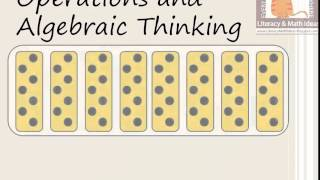By Literarcy and Math ideas

Quotient of whole numbers# Estimating Quotients | MathHelp.com

##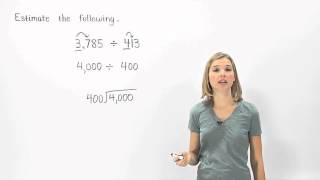By MathHelp.com

This lesson covers commutative property of multiplication, which states that a x b = b x a. For example, 10 x 4 = 4 x 10.# Quotient Rule | Dividing with Exponents | MathHelp.com

##By MathHelp.com# Imaginary Numbers | MathHelp.com

##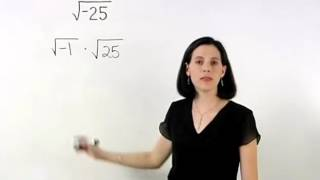By MathHelp.com

This lesson covers estimating quotients. Students learn to estimate a product or quotient by first rounding each number to one non-zero digit. For example, to estimate 8,291 x 27, first round 8,291 down to 8,000, and round 27 up to 30, then multiply 8,000 x 30 to get 240,000.# [4.NBT.6-1.0] Four digit division - Common Core Standard

##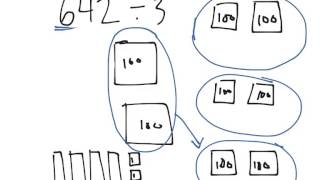By Freckle education

Find whole-number quotients and remainders with up to four-digit dividends and one-digit divisors, using strategies based on place value, the properties of operations, and/or the relationship between multiplication and division# 8th Grade Math | MathHelp.com

##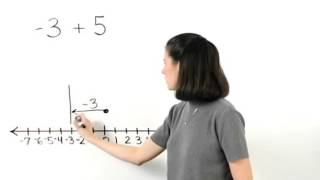By MathHelp.com

This lesson covers estimating products. Students learn to estimate a product or quotient by first rounding each number to one non-zero digit. For example, to estimate 8,291 x 27, first round 8,291 down to 8,000, and round 27 up to 30, then multiply 8,000 x 30 to get 240,000.# Multiplying and Dividing Decimals | 7.NS.A.2c | 7th Grade Math

##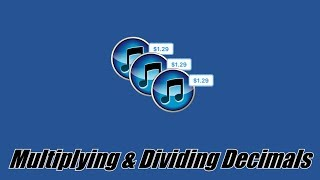By ParksMath | Todd Parks

ParksMath explains how to multiply and divide decimals numbers without the use of a calculator. Understanding how to change a decimal number so that it is an integer, makes multiplying and dividing decimals much easier. This quick tutorial will give you thee tools that you need to find the product or quotient of any decimal number.# Multiplying and Dividing Decimals | 7.NS.A.2c | 7th Grade Math

##By ParksMath

ParksMath explains how to multiply and divide decimals numbers without the use of a calculator. Understanding how to change a decimal number so that it is an integer, makes multiplying and dividing decimals much easier. This quick tutorial will give you thee tools that you need to find the product or quotient of any decimal number.# Congruent Figures | MathHelp.com

##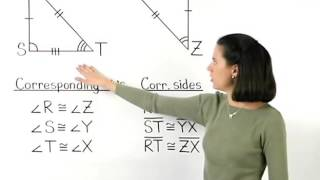By MathHelp.com

This lesson covers dividing integers. Students learn to divide integers using the following rules. A positive divided by a positive equals a positive. For example, +20 divided by +2 = +10. A positive divided by a negative equals a negative. For example, +20 divided by -2 = -10. A negative divided by a positive equals a negative. For example, -20 divided by +2 = -10. And a negative divided by a negative equals a positive. For example, -20 divided by -2 = +10. In other words, if the signs are the same, the quotient is positive, and if the signs are different, the quotient is negative. Note that any integer divided by zero is undefined. For example, +4 divided by 0 = undefined. And zero divided by any integer (other than zero) is zero. For example, 0 divided by +4 = 0.# [7.NS.2b-1.0] Division of Signed Numbers - Common Core Standard

##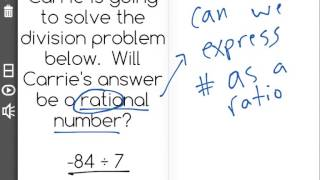By Freckle by Renaissance

Understand that integers can be divided, provided that the divisor is not zero, and every quotient of integers (with non-zero divisor) is a rational number. Front Row is a free, adaptive, Common Core aligned math program for teachers and students in kindergarten through eighth grade. Front Row allows students to practice math at their own pace - learning advanced concepts when they're ready and receiving remediation when they struggle. Front Row provides teachers with access to a detailed data dashboard and weekly email reports that show which standards are causing students difficulty, what small groups can be formed for interventions, and how their students are progressing in math.# Simplifying a radical expression using rational exponents

##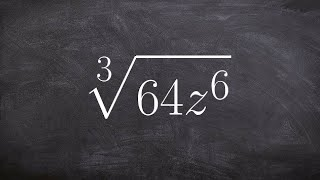By Brain McLogan

Learn how to simplify rational powers using the power and the product rules. There are some laws of exponents which might come handy when simplifying expressions with exponents. Some of the laws include the product law which states that the product of numbers/expressions having the same base is equivalent to taking one of the base with the sum of the original exponents as the new exponent. The power law says that when an expression with an exponent is raised to another exponent that it is equivalent to the expression with the product of the original exponents as the new exponent. Knowledge of these exponent laws will help you in simplifying power expressions using the power rule and the quotient rule.# [5.NBT.6-1.0] Multi-digit divisors - Common Core Standard - Word Problem

##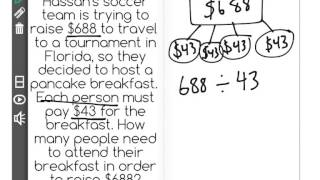By Front Row

Discover more Common Core Math at https://www.frontrowed.com Find whole-number quotients of whole numbers with up to four-digit dividends and two-digit divisors, using strategies based on place value, the properties of operations, and/or the relationship between multiplication and division Front Row is a free, adaptive, Common Core aligned math program for teachers and students in kindergarten through eighth grade. Front Row allows students to practice math at their own pace - learning advanced concepts when they're ready and receiving remediation when they struggle. Front Row provides teachers with access to a detailed data dashboard and weekly email reports that show which standards are causing students difficulty, what small groups can be formed for interventions, and how their students are progressing in math.Discover more Common Core Math at https://www.frontrowed.com# Learn Division for Kids - 2nd and 3rd Grade Math Video

##By Lumos Learning

Check out the NEW Math Game we made at https://www.MageMath.com/​ It is a full video game called Mage Math that helps kids build confidence in math while having tons of fun.# [3.OA.2-1.0] Basic Division - Common Core Standard - Word Problem

##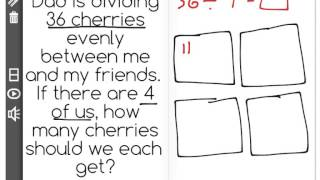By Front Row

Discover more Common Core Math at https://www.frontrowed.comInterpret whole-number quotients of whole numbers, e.g., interpret 56 ÃƒÂ· 8 as the number of objects in each share when 56 objects are partitioned equally into 8 shares, or as a number of shares when 56 objects are partitioned into equal shares of 8 objects each. For example, describe a context in which a number of shares or a number of groups can be expressed as 56 ÃƒÂ· 8.Front Row is a free, adaptive, Common Core aligned math program for teachers and students in kindergarten through eighth grade. Front Row allows students to practice math at their own pace - learning advanced concepts when they 're ready and receiving remediation when they struggle. Front Row provides teachers with access to a detailed data dashboard and weekly email reports that show which standards are causing students difficulty, what small groups can be formed for interventions, and how their students are progressing in math.Discover more Common Core Math at https://www.frontrowed.com# Evaluating Logarithms | MathHelp.com

##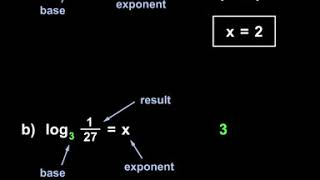By MathHelp.com

In this example, notice that we have a polynomial divided by a binomial, and our binomial is in the form of an x term minus a constant term, or x – c. In this situation, instead of having to use long division, like we did in the previous lesson, we can divide the polynomials using synthetic division, which is a much more efficient method. Here’s how it works. We start by finding the value of c. Since –c = -3, we know that c = 3. Next, we put the value of c inside a box, so we put the 3 inside a box. It’s very important to understand that the number that goes inside the box always uses the opposite sign as the constant term in the binomial. In other words, since the constant term in the binomial is -3, the number that goes inside the box, is positive 3. Next, we write the coefficients of the dividend, which are 2, -7, 4, and 5. Be very careful with your signs. Now, we’re ready to start our synthetic division. First, we bring down the 2. Next, we multiply the 3 in the box times 2 to get 6, and we put the 6 under the -7. Next, we add -7 + 6 to get -1. Next, we multiply the 3 in the box times -1 to get -3, and we put the -3 under the 4. Next, we add 4 + -3 to get 1. Next, we multiply the 3 in the box times 1 to get 3, and we put the 3 under the 5. Finally, we add 5 + 3 to get 8. Now, notice that we have a 2, -1, 1, and 8 in the bottom row of our synthetic division. These values will give us our answer: the first 3 numbers represent the coefficients of the quotient, and the last number is the remainder. And it’s important to understand that our answer will be one degree less than the dividend. In other words, since our dividend starts with x cubed, and we’re dividing by x, our answer will start with x squared. So our answer is 2x squared – 1x + 1 + 8 over x – 3. Notice that we always use descending order of powers in our quotient. In this case x squared, x, and the constant. Finally, remember that we add the remainder over the divisor, just like we did in the previous lesson on long division, and we have our answer. It’s important to understand that we’ll get the same answer whether we use synthetic division or long division. However, synthetic division is much faster.# Exponents | MathHelp.com

##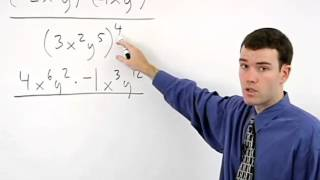By MathHelp.com